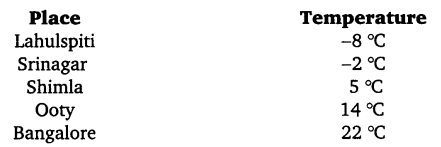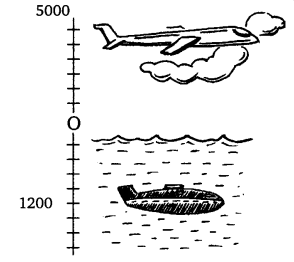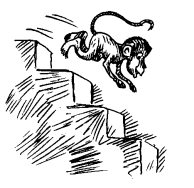# Class 7 Maths NCERT Solutions for Chapter 1 Integers Chapter 1 Integers EX – 1.1

## Integers

Question 1.
Following number line shows the temperature in degree Celsius (°C) at different places on a particular day.(a) Observe this number line and write the temperature of the places marked on it.
(b) What is the temperature difference between the hottest and the coldest places among the above?
(c) What is the temperature difference between Lahulspiti and Srinagar?
(d) Can we say temperature of Srinagar and Shimla taken together is less than the temperature at Shimla? Is it also less than the temperature at Srinagar?

(a) From the given number line, we find that the temperature of the indicated places as under :(b) The hottest place is Bangalore (22 °C) and the coldest place is Lahulspiti (-8°C). The temperature difference between the hottest and the coldest places
= 22 °C – (-8 °C)
= 22 °C +8 °C = 30 °C
(c) The temperature difference between Lahulspiti and Srinagar
= -2 °C – (-8 °C)
= -2 °C +8 °C = 6 °C
(d) Yes, we can say that the temperature of Srinagar and Shimla taken together is less than the temperature at Shimla as -2 + 5 = 3 and 3 < 5. This temperature is not less than the temperature at Srinagar.

Question 2.
In a quiz, positive marks are given for correct answers and negative marks are given for incorrect answers. If Jack’s scores in five successive rounds were 25, 5, -10, 15 and 10, what was his total at the end?

Solution.
Jack’s scores in five successive rounds were given as 25, -5, -10, 15 and 10.
Jack’s total score
=25 +(-5)+ (-10)+ 15+ 10
= 25-5-10+15 + 10 = 50-15 = 35

Question 3.
At Srinagar, temperature was -5 °C on Monday and then it dropped by 2 C on Tuesday. What was the temperature of Srinagar on Tuesday? On Wednesday, it rose by 4 °C. What was the temperature on this day?

Solution.
At Srinagar, temperature was on Monday = -5 °C
Since, the temperature was dropped by 2 °C on Tuesday, therefore, temperature was on Tuesday = (-5-2) °C = -7°C
Also, on Wednesday the temperature rose by 4 °C.
∴ Temperature on Wednesday= (-7 + 4) °C = -3 °C

Question 4.
A plane is flying at the height of 5000 m above the sea level. At a particular point, it is exactly above a submarine floating 1200 m below the sea level. What is the vertical distance between them?Solution.
Vertical distance between the plane and the submarine
= 5000 m + 1200 m = 6200 mQuestion 5.
Mohan deposits ₹ 2,000 in his bank account and withdraws ₹ 1,642 from it, the next day. If withdrawal of amount from the account is represented by a negative integer, then how will you represent the amount deposited? Find the balance in Mohan’s account after the withdrawal.

Solution.
Amount deposited will be represented by a positive integer.
Balance in Mohan’s account after withdrawal
= (+ ₹ 2000) + (- ₹ 1642)
= ₹ (2000-1642)
= ₹ 358

Question 6.
Rita goes 20 km towards east from a point A to the point B. From B, she moves 30 km towards west along the same road. If the distance towards east is represented by a positive integer, then how will you represent the distance travelled towards west? By which integer will you represent her final position from A?Solution.
The distance towards west will be represented by a negative integer.
Rita’s movement is shown as under :Since, Rita moves 20 km towards east from a point A, so she reaches B and then from B she moves 30 km towards west along the same road and reaches C.
Thus, her final position from A will be represented by the integer -10.

Question 7.
In a magic square each row, column and diagonal have the same sum. Check, which of the following is a magic square?Solution.
In square (i) :
Row 1 : 5 + (-1) + (-4) = 5-1-4 =0
Row 2 : (-5) + (-2) + 7 =-5-2 + 7 = 0
Row 3 : 0 + 3 + (-3) =0+3-3 =0
Column 1 : 5 + (-5) + 0= 5- 5 + 0= 0
Column 2 : (-1) + (-2) + 3 = -1 -2 + 3 = 0
Column 3 : (-4) + 7 + (-3) = -4 + 7- 3 = 0
Diagonal 1 : 5 + (-2) + (-3) = 5 – 2 – 3 =0
Diagonal 2 : (-4) + (-2) + 0 = -4 – 2 + 0 = -6
∵ The sum of digits along the diagonal 2 ≠ 0.
Thus, it is not a magic square.
In square (ii) :
Row 1 : 1 + (-10) + 0 = 1-10+0 = -9
Row 2 : (-4) +(-3) +(-2) = -4-3-2 = -9
Row 3 : (-6) + 4 + (-7) = -6 + 4 – 7 = -9
Column 1 : 1 + (-4) + (-6) = 1- 4- 6 = -9
Column 2 : (-10) + (-3) + 4 = -10-3 + 4 = -9
Column 3 : 0 + (-2) + (-7) = 0-2-7 =-9
Diagonal 1 : 1 + (-3) + (-7) = -9
Diagonal 2: 0 + (-3) + (-6) = 0- 3- 6 = -9
∵ Each row, column and diagonal have the same sum.
Thus, it is a magic square.

Question 8.
Verify a – (-b) = a + b for the following values of a and b:

1. a = 21, b = 18
2. a = 118, b = 125
3. a = 75, b = 84
4. a = 28, b = 11

Solution.

1. L.H.S. = a – (-b) = 21 – (-18) = 21 +18 = 39
R.H.S. = a + b = 21 +18 =39
∴ L.H.S. = R.H.S.
2.  L.H.S. = a – (-b) = 118 – (-125) = 118 +125 = 243
R.H.S. = a + b = 118+125 = 243
∴ L.H.S. = R.H.S.
3.  L.H.S. = a – (-b) = 75 – (-84) = 75+ 84 = 159
R.H.S. = a + b =75 + 84 = 159
4.  L.H.S. = a – (-b) = 28 – (-11) = 28+ 11 = 39
R.H.S. = a + b = 28 + 11 = 39

Question 9.
Use the sign of >, < or = in the box to make the statements true.
(a)
(-8) + (-4)$\boxed { }$ (-8) – (-4)
(b) (-3) + 7 – (19)$\boxed { }$ 15 – 8 +(-9)
(c) 23 – 41 + 11$\boxed { }$ 23 – 41 – 11
(d) 39+ (-24) – (15)$\boxed { }$ 36 + (-52) – (-36)
(e) -231 + 79 + 51$\boxed { }$ -399 + 159 + 81

Solution.Question 10.
A water tank has steps inside it. A monkey is sitting on the topmost step (i.e., the first step). The water level is at the ninth step.
(i)
He jumps 3 steps down and then jumps back 2 steps up. In how many jumps will he reach the water level?
(ii) After drinking water, he wants to go back. For this, he jumps 4 steps up and then jumps back 2 steps down in every move. In how many jumps will he reach back the top step?(iii) If the number of steps moved down is represented by negative integers and the number of steps moved up by positive integers, represent his moves in part (i) and (ii) by completing the following :
(a) -3 + 2-… = -8
(b) 4-2 +… = 8.
In (a), the sum (-8) represents going down by eight steps. So, what will the sum 8 in (b) represent?

Solution.
(i) While going down the monkey jumps 3 steps down and then jumps back 2 steps up. To reach the water level he is to jump as under:
-3+ 2 -3 + 2 – 3 +2 – 3 + 2 – 3 + 2 – 3 = -8 Hence, he takes 11 jumps to reach the water level.
(ii) After drinking water, he jumps back as under to reach the top step as under : 4 – 2+ 4 – 2+ 4 = 8
Hence, he takes 5 jumps to reach back the top.
(iii) (a) – 3 + 2 – 3 + 2 – 3 + 2 – 3 + 2 – 3 + 2 – 3 = -8
(b) 4 – 2 + 4 – 2 + 4 = 8
In (b) the sum 8 represents going up 8 steps.